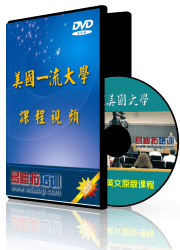# Structure and Interpretation of Systems and Signal

### EE401：信号与系统基础视频课程

Instructor: Prof. Babak Ayazifar

UC Berkeley 本科生专业课

Structure and Interpretation of Systems and Signals 是是UC Berkekey本科生课程 ，是信号与系统相关课程基础的入门课程，共28讲，每讲75分钟左右。课程视频全为.rm 格式，同时有与视频课程内容配套的部分课程讲义；有了课程讲义让您的学习更有效，在潜移默化中提高专业知识和英语能力。

Course Description

This course provides an accessible introduction to signals and systems for electrical engineering, computer engineering, and computer science students. Here introduces mathematical modeling techniques used in the design of electronic systems. An important keyword here is "mathematical."

Signals are defined as functions on respective sets. Examples include:

● Continuous-time signals (audio, radio, voltages);

● Discrete-time signals (digital audio, synchronous circuits);

● Images (discrete and continuous);

● Discrete-event signals;

● Sequences.

Systems are defined as mappings on signals. The notion of the state is discussed in a general way. Feedback systems and automata illustrate alternative approaches to modeling state in systems.

Automata theory is studied using Mealy machines with input and output. Notions of equivalence of automata and concurrent composition are introduced.

Hybrid systems combine time-based signals with event sequences.

Difference and differential equations are considered as models for linear, time-invariant state machines.

Frequency domain models for signals and frequency response for systems are investigated.

Sampling of continuous signals is discussed to relate continuous time and discrete time signals.

Applications include communications systems, audio, video, and image processing systems, and control systems.

A MATLAB-based laboratory is an integral part of the course.

Textbooks

E. A. Lee and P. Varaiya, Structure and Interpretation of Signals and Systems, Addison-Wesley, 2003.

Course Objectives

This course introduces mathematical modeling techniques used in the study of signals and systems. Its intention is to promote rigorous thinking and mathematical intuition about, and an appreciation for a multidisciplinary study of, signals systems through precise modeling.全8门视频课程,普通话讲解,企业员工内训课,讲解多从工程实践出发，射频/通信工程师经典学习教程,值得推荐【More..

网站地图